# Graphing Linear Equations Identifying a Linear Equation A

• Slides: 9Graphing Linear EquationsIdentifying a Linear Equation A linear equation is any equation that can be put in the form. . . Ax + By = C. . . where A, B, and C are real numbers, and A and B are not both zero. The graph of a linear equation is a line. Which of the following equations are linear equations? 3 x + y = 8 2(x + 3)(x + 4) = y 2 x 2 + 3 x + 4 = y 5 y + x = 1 – 3 x 2 – y = 9 8 x – 3 x + x – 4 = y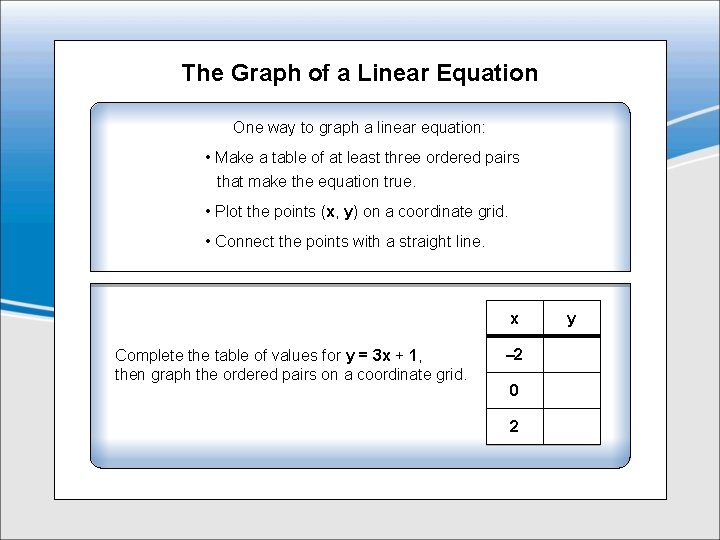The Graph of a Linear Equation One way to graph a linear equation: • Make a table of at least three ordered pairs that make the equation true. • Plot the points (x, y) on a coordinate grid. • Connect the points with a straight line. x Complete the table of values for y = 3 x + 1, then graph the ordered pairs on a coordinate grid. – 2 0 2 y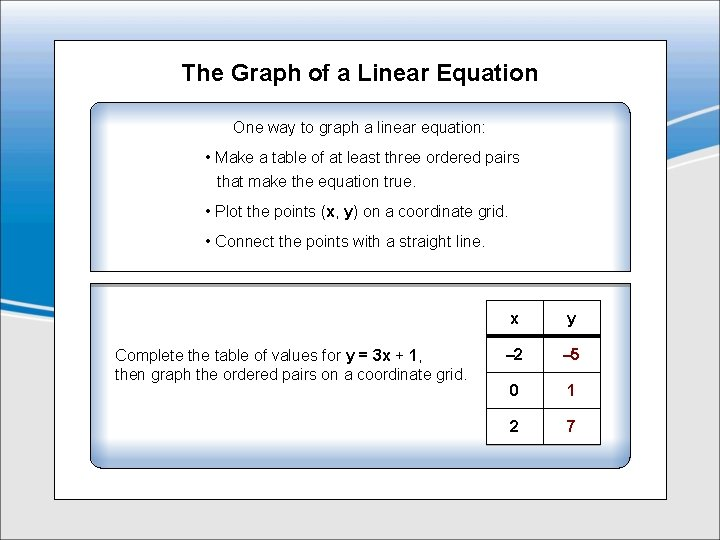The Graph of a Linear Equation One way to graph a linear equation: • Make a table of at least three ordered pairs that make the equation true. • Plot the points (x, y) on a coordinate grid. • Connect the points with a straight line. Complete the table of values for y = 3 x + 1, then graph the ordered pairs on a coordinate grid. x y – 2 – 5 0 1 2 7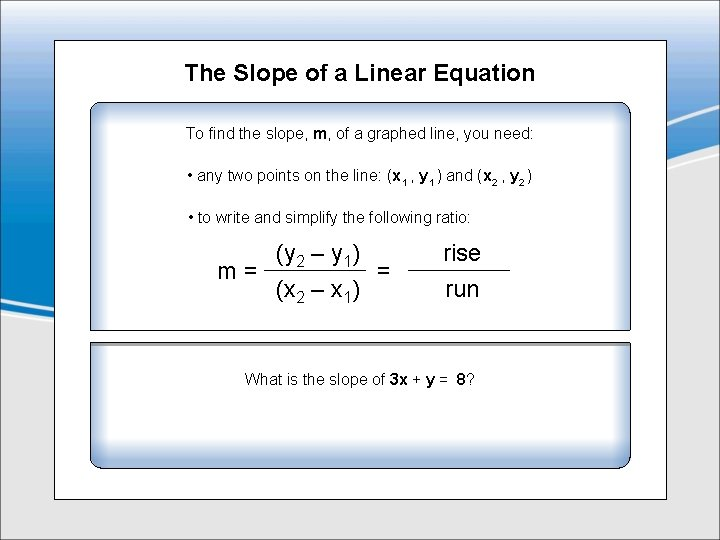The Slope of a Linear Equation To find the slope, m, of a graphed line, you need: • any two points on the line: (x 1 , y 1 ) and (x 2 , y 2 ) • to write and simplify the following ratio: (y 2 – y 1) m= = (x 2 – x 1) rise run What is the slope of 3 x + y = 8?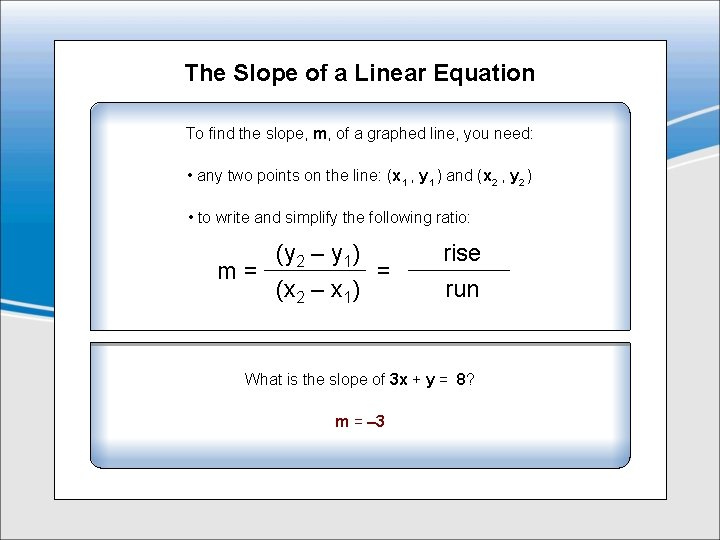The Slope of a Linear Equation To find the slope, m, of a graphed line, you need: • any two points on the line: (x 1 , y 1 ) and (x 2 , y 2 ) • to write and simplify the following ratio: (y 2 – y 1) m= = (x 2 – x 1) rise run What is the slope of 3 x + y = 8? m = – 3Forms of a Linear Equation Slope-Intercept Form: y = mx + b, where m is the slope and b is the y-intercept Point-Slope Form: y – y 1 = m(x – x 1 ), where m is the slope and x 1 and y 1 are the coordinates for any point on the line Write y – 1 = 3 x in Slope-Intercept form. Find the slope and the y-intercept. Use the Point-Slope form to write the equation for the line that passes through points (1, 3) and (– 11, – 5) in Ax + By = C form.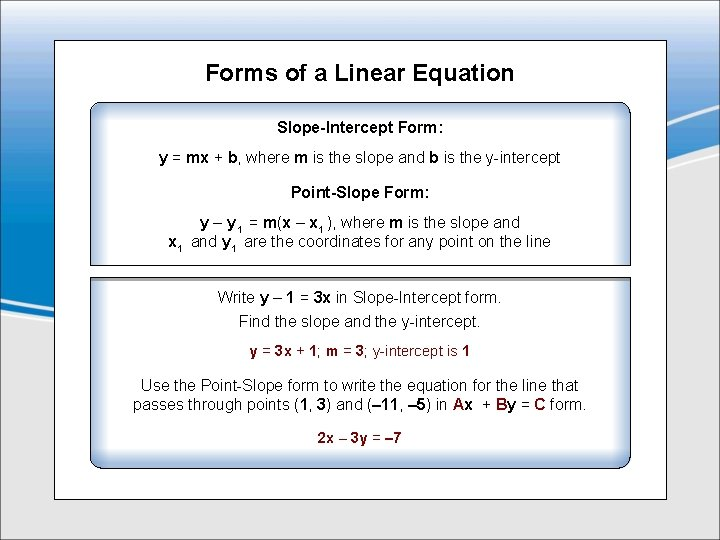Forms of a Linear Equation Slope-Intercept Form: y = mx + b, where m is the slope and b is the y-intercept Point-Slope Form: y – y 1 = m(x – x 1 ), where m is the slope and x 1 and y 1 are the coordinates for any point on the line Write y – 1 = 3 x in Slope-Intercept form. Find the slope and the y-intercept. y = 3 x + 1; m = 3; y-intercept is 1 Use the Point-Slope form to write the equation for the line that passes through points (1, 3) and (– 11, – 5) in Ax + By = C form. 2 x – 3 y = – 7